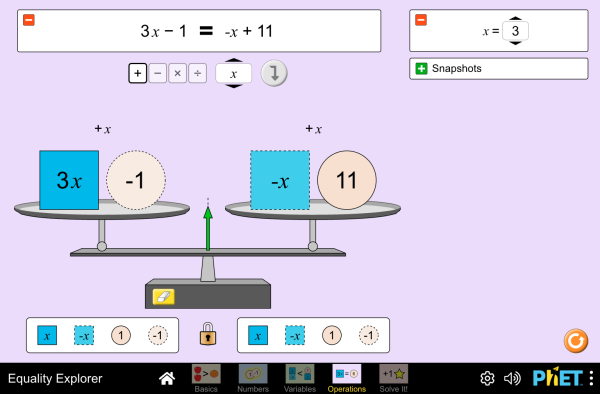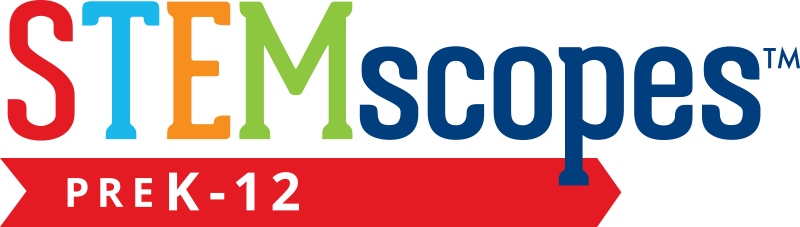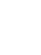# Equality Explorer

Aceste simulări nu au fost încă traduse în limba română . Puteți accesa simularea, din meniul de mai jos, în limba engleză.DescarcăInclude Închide Include o copie functională a simulării Folosiţi acest cod sursă pentru a include o simulare. Puteţi mofifica dimensiunile prin modificarea parametrilor "width" şi "height". Include o imagine care lansează simularea
Rulează
Folosiţi acest cod sursă pentru afişarea "Rulează" Solving Equations Inequalities Inverse Operations PhET is supported byand educators like you.

### Subiecte

• Solving Equations
• Inequalities
• Inverse Operations

### Descriere

Explore what it means for a mathematical statement to be balanced or unbalanced by interacting with objects on a balance. Discover the rules for keeping it balanced. Collect stars by playing the game!

### Studii de caz

• Solve and manipulate algebraic equations by applying properties of real numbers (in particular, the inverse properties of addition and multiplication) and properties of equality
• Solve and manipulate algebraic equations by substituting different values for a variable
• Use a balance model to solve an equation for an unknown, and justify your strategies for solving
• Solve an equation using only universal operations

### Standards Alignment

#### Common Core - Math

6.EE.B.5
Understand solving an equation or inequality as a process of answering a question: which values from a specified set, if any, make the equation or inequality true? Use substitution to determine whether a given number in a specified set makes an equation or inequality true.
6.EE.B.6
Use variables to represent numbers and write expressions when solving a real-world or mathematical problem; understand that a variable can represent an unknown number, or, depending on the purpose at hand, any number in a specified set.
7.EE.B.3
Solve multi-step real-life and mathematical problems posed with positive and negative rational numbers in any form (whole numbers, fractions, and decimals), using tools strategically. Apply properties of operations to calculate with numbers in any form; convert between forms as appropriate; and assess the reasonableness of answers using mental computation and estimation strategies. For example: If a woman making \$25 an hour gets a 10% raise, she will make an additional 1/10 of her salary an hour, or \$2.50, for a new salary of \$27.50. If you want to place a towel bar 9 3/4 inches long in the center of a door that is 27 1/2 inches wide, you will need to place the bar about 9 inches from each edge; this estimate can be used as a check on the exact computation.
7.EE.B.4
Use variables to represent quantities in a real-world or mathematical problem, and construct simple equations and inequalities to solve problems by reasoning about the quantities.
8.EE.C.7
Solve linear equations in one variable.
8.EE.C.7a
Give examples of linear equations in one variable with one solution, infinitely many solutions, or no solutions. Show which of these possibilities is the case by successively transforming the given equation into simpler forms, until an equivalent equation of the form x = a, a = a, or a = b results (where a and b are different numbers).
8.EE.C.8
Analyze and solve pairs of simultaneous linear equations.
HSA-CED.A.1
Create equations and inequalities in one variable and use them to solve problems. Include equations arising from linear and quadratic functions, and simple rational and exponential functions.
HSA-CED.A.3
Represent constraints by equations or inequalities, and by systems of equations and/or inequalities, and interpret solutions as viable or nonviable options in a modeling context. For example, represent inequalities describing nutritional and cost constraints on combinations of different foods.
HSA-REI.A.1
Explain each step in solving a simple equation as following from the equality of numbers asserted at the previous step, starting from the assumption that the original equation has a solution. Construct a viable argument to justify a solution method.
HSA-REI.B.3
Solve linear equations and inequalities in one variable, including equations with coefficients represented by letters.
Versiune 1.0.12

### Sfaturi pentru profesoriGhidul profesorului conține un sumar al elementelor de control ale simulării, modelul ideal, perspective asupra gândirii elevilor ( PDF ).

### Activități ale profesorilor

Games Remote Lesson ideasTrish Loeblein K-5
HS
MS
UG-Intro
Remote
HW
Physics
Mathematics
Chemistry
Introducción a la igualdadDiana López MS
HS
Lab
HW
Guided
Mathematics
Balancing ActMichael Reynolds MS Guided Mathematics
What is EqualityDavid Wood MS
HS
Discuss
Lab
Guided
HW
Mathematics
Introduction to InequalitiesMarleene Buttice MS Guided Mathematics
Solving Multi-Step EquationsMeenakshi Raghavan MS Guided Mathematics
Middle School Math Sim AlignmentAmanda McGarry MS Other Mathematics
SECUNDARIA: Alineación PhET con programas de la SEP México (2011 y 2017)Diana López MS
HS
Other Chemistry
Biology
Mathematics
Physics
PREPARATORIA: Alineación de PhET con programas de la DGB México (2017)Diana López UG-Intro
HS
Other Chemistry
Mathematics
Physics
PRIMARIA: Alineación con programas de la SEP México (2011 y 2017)Diana López MS
K-5
Lab
Guided
HW
Discuss
Demo
Chemistry
Mathematics
Physics
Astronomy
Equality Explorer - 6th Grade intro to solving Trevor Gibson MS Guided Mathematics
Exploring Equations Akili Obika MS
HS
Guided Mathematics
Equação e balança Alison Sousa da Silva, Ana Carolina Costa Pereira MS Guided
Lab
Mathematics
Limba Downloador RunTips
Albanian All shqipHulumtues barazishë
Arabic All العربيةمستكشف المساواة
Basque All EuskaraBerdintasun-esploratzailea
Bosnian All BosanskiIstraživač jednačina
Brazilian Portuguese All português (Brasil)Explorador da Igualdade
Chinese - Simplified All 中文 (中国)等式探索
Chinese - Traditional All 中文 (台灣)Equality Explorer_探索等式
Croatian All hrvatskiIstraživanje jednadžbi
Czech All češtinaPrůzkumník rovnosti
Danish All DanskLigevægts-visning
Dutch All NederlandsOntdek gelijkheid
French All françaisExplorateur d'égalité
German All DeutschGleichheit schaffen
Greek All ΕλληνικάΕξερευνητής εξισώσεων
Gujarati All Gujaratiસમીકરણ સમાનતા શોધક- Pankajsid34
Hindi All हिंदीसमानता का पता करें
Hungarian All magyarEgyenlőségvizsgálat
Italian All italianoEsplora le equazioni
Japanese All 日本語つり合いを作ろう
Kazakh All KazakhТеңсіздіктер шешімі
Korean All 한국어등식 연습하기
Latvian All LatviešuVienādību pētīšana
Mongolian All Монгол (Монгол)Тэнцэтгэл Судлах
Persian All فارسیکاوشگر برابری
Polish All polskiPoznajemy równania
Portuguese All portuguêsExplorador de Igualdades
Russian All русскийРешение неравенств
Serbian All СрпскиИстраживач једначина
Slovak All SlovenčinaSkúmanie rovnosti
Spanish All españolExplorador de IgualdadesSpanish (Mexico) All español (México)Explorador de IgualdadesSpanish (Peru) All español (Perú)Explorador de Igualdad
Swedish All svenskaUtforska likheter!
Turkish All TürkçeEşitlik Gezgini
Ukrainian All українськаДослідник рівностейVietnamese All Tiếng ViệtPhương trình

HTML5 sims can run on iPads and Chromebooks, as well as PC, Mac, and Linux systems.

iOS 12+ Safari

Android:
Not officially supported. If you are using the HTML5 sims on Android, we recommend using the latest version of Google Chrome.

Chromebook:
The HTML5 and Flash PhET sims are supported on all Chromebooks.
Chromebook compatible sims

Windows Systems:

Macintosh Systems:

Linux Systems:

Echipa de proiectare Alţi colaboratori Mulţumiri
• Chris Malley (developer)
• Diana López Tavares
• Ariel Paul
• Kathy Perkins
• Argenta Price
• David Webb
• Mariah Hermsmeyer (graphic arts)
• Cheryl McCutchan (graphic arts)
• almond-0.2.9.js
• base64-js-1.2.0.js
• easing-equations-r12
• FileSaver-b8054a2.js
• font-awesome-4.5.0
• game-up-camera-1.0.0.js
• he-1.1.1.js
• himalaya-0.2.7.js
• jama-1.0.2
• jquery-2.1.0.js
• lodash-4.17.4.js
• pegjs-0.7.0.js
• seedrandom-2.4.2.js
• text-2.0.12.js
• TextEncoderLite-3c9f6f0.js
• Tween-r12.js